# Excel Formulas

## Formulas

A formula in Excel is used to do mathematical calculations. Formulas always start with the equal sign (`=`) typed in the cell, followed by your calculation.

Formulas can be used for calculations such as:

• `=1+1`
• `=2*2`
• `=4/2=2`

It can also be used to calculate values using cells as input.

Let's have a look at an example.

Type or copy the following values: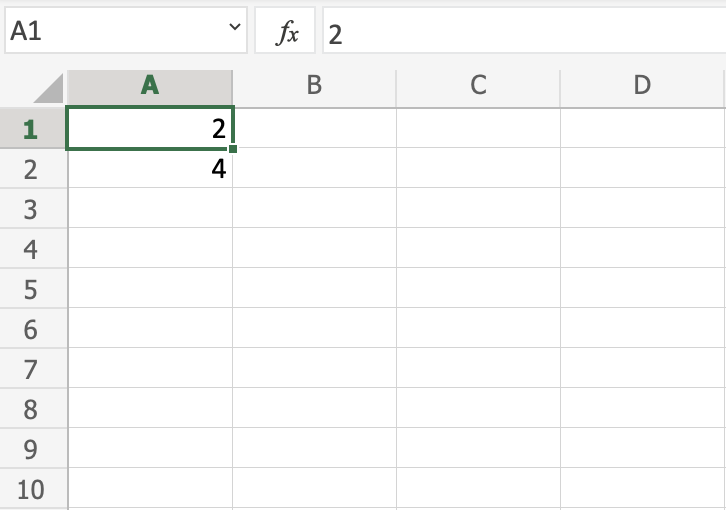Copy Values

Now we want to do a calculation with those values.

Step by step:

1. Select `C1` and type (`=`)
2. Left click `A1`
3. Type (`+`)
4. Left click `A2`
5. Press enter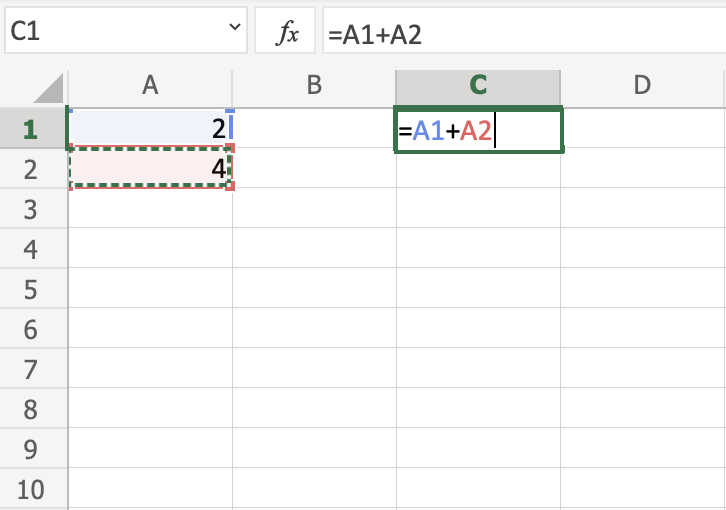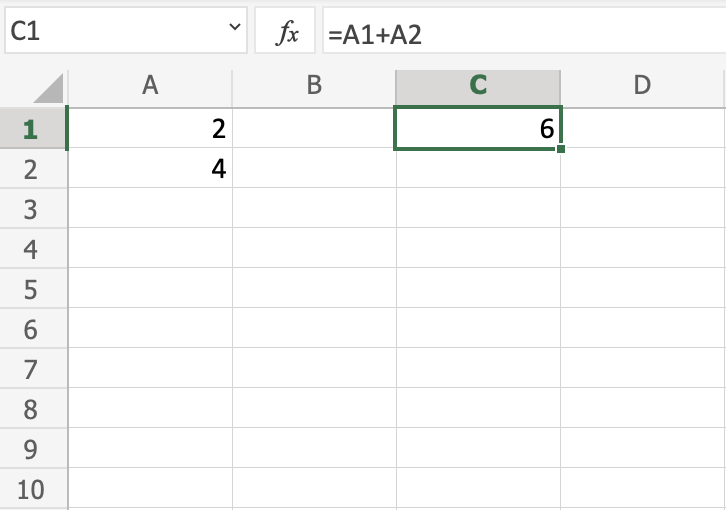You got it! You have successfully calculated `A1(2) + A2(4) = C1(6)`.

Note: Using cells to make calculations is an important part of Excel and you will use this alot as you learn.

Lets change from addition to multiplication, by replacing the (+) with a (*). It should now be `=A1*A2`, press enter to see what happens.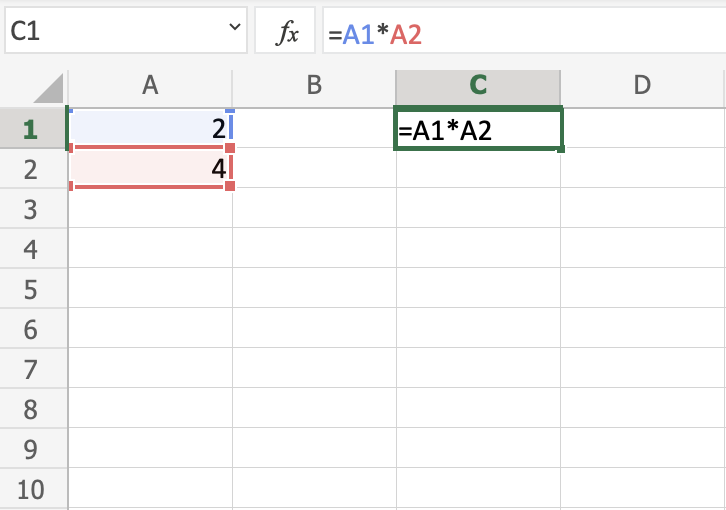You got `C1(8)`, right? Well done!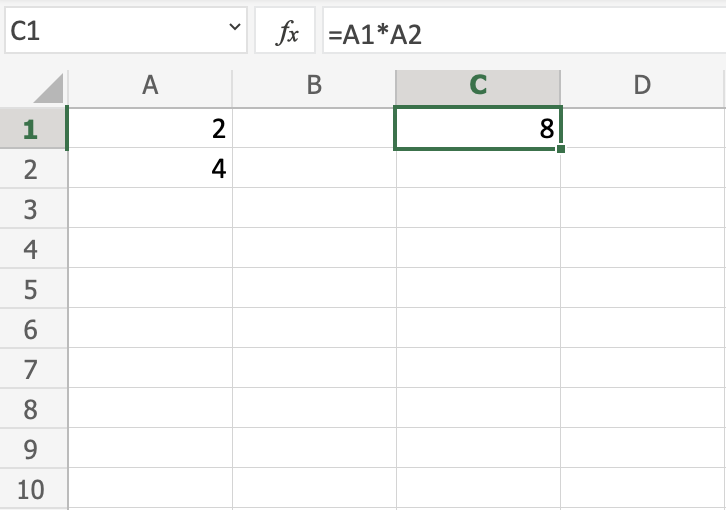Excel is great in this way. It allows you to add values to cells and make you do calculations on them.

Now, try to change the multiplication (`*`) to subtraction (`-`) and dividing (`/`).

Delete all values in the sheet after you have tried the different combinations.

Let's add new data for the next example, where we will help the Pokemon trainers to count their Pokeballs.

Type or copy the following values: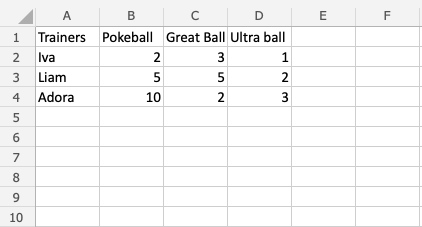Copy Values

The data explained:

• Column `A`: Pokemon Trainers
• Row `1`: Types of Pokeballs
• Range `B2:D4`: Amount of Pokeballs, Great balls and Ultra balls

Note: It is important to practice reading data to understand its context. In this example you should focus on the trainers and their Pokeballs, which have three different types: Pokeball, Great ball and Ultra ball.

Let's help Iva to count her Pokeballs. You find Iva in `A2(Iva)`. The values in `row 2 B2(2), C2(3), D2(1)` belong to her.

Count the Pokeballs, step by step:

1. Select cell `E2` and type (`=`)
2. Left click `B2`
3. Type (`+`)
4. Left click `C2`
5. Type (`+`)
6. Left click `D2`
7. Hit enter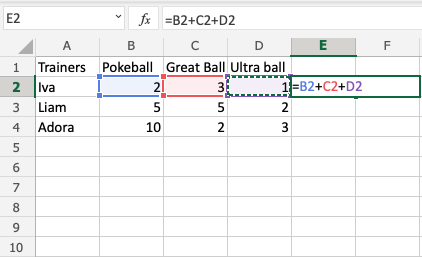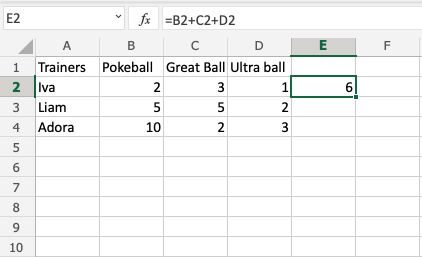Did you get the value `E2(6)`? Good job! You have helped Iva to count her Pokeballs.

Now, let's help Liam and Adora with counting theirs.

Do you remember the fill function that we learned about earlier? It can be used to continue calculations sidewards, downwards and upwards. Let's try it!

Lets use the fill function to continue the formula, step by step:

1. Select `E2`
2. Fill `E2:E4`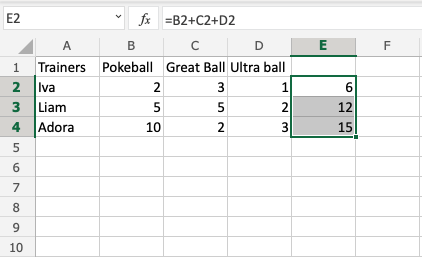That is cool, right? The fill function continued the calculation that you used for Iva and was able to understand that you wanted to count the cells in the next rows as well.

Now we have counted the Pokeballs for all three; Iva(`6`), Liam(`12`) and Adora(`15`).

Let's see how many Pokeballs Iva, Liam and Adora have in total.

The total is called SUM in Excel.

There are two ways to calculate the SUM.

• SUM function

Excel has many pre-made functions available for you to use. The SUM function is one of the most used ones. You will learn more about functions in a later chapter.

Let's try both approaches.

Note: You can navigate to the cells with your keyboard arrows instead of left clicking them. Try it!

Sum by adding cells, step by step:

1. Select cell E5, and type `=`
2. Left click `E2`
3. Type (`+`)
4. Left click `E3`
5. Type (`+`)
6. Left click `E4`
7. Hit enter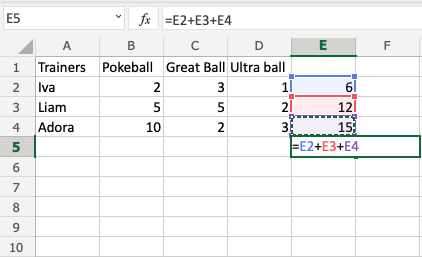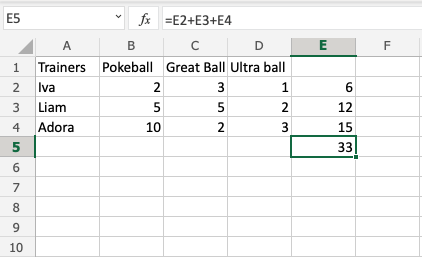The result is `E5(33)`.

Let's try the SUM function.

Remember to delete the values that you currently have in `E5`.

SUM function, step by step:

1. Type `E5(=)`
2. Write SUM
3. Double click SUM in the menu
4. Mark the range `E2:E4`
5. Hit enter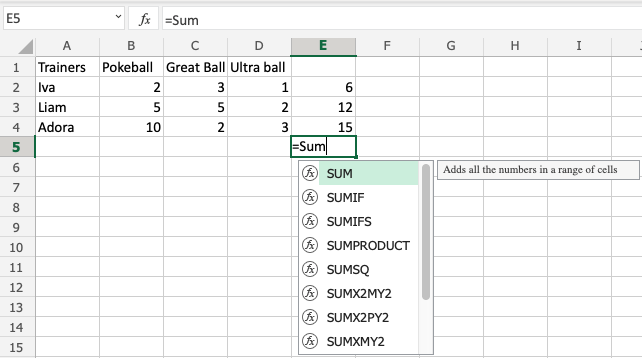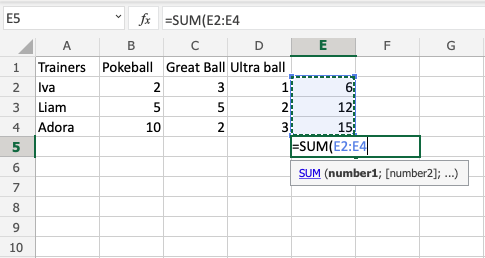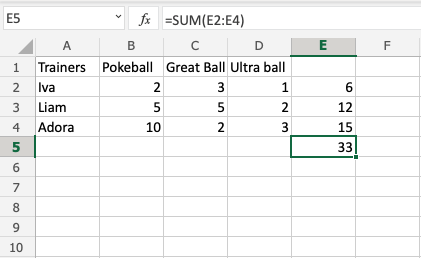Great job! You have successfully calculated the SUM using the SUM function.

Iva, Liam and Adora have `33` Pokeballs in total.

Let's change a value to see what happens. Type `B2(7)`: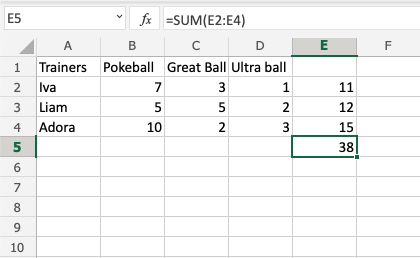The value in cell `B2` was changed from `2` to `7`. Notice that the formulas are doing calculations when we change the value in the cells, and the SUM is updated from `33` to `38`. It allows us to change values that are used by the formulas, and the calculations remain.

## Chapter Summary

Values used in formulas can be typed directly and by using cells. The formula updates the result if you change the value of cells, which is used in the formula. The fill function can be used to continue your formulas upwards, downwards and sidewards. Excel has pre-built functions, such as SUM.

In the next chapter you will learn about relative and absolute references.

## Excel Exercise:

Complete the Excel formula:

```8+10
```

Start the Exercise

W3Schools is optimized for learning and training. Examples might be simplified to improve reading and learning. Tutorials, references, and examples are constantly reviewed to avoid errors, but we cannot warrant full correctness of all content. While using W3Schools, you agree to have read and accepted our terms of use, cookie and privacy policy.